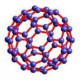Installation using Arduino

I am doing an Installtion using Arduino connected to SuperCollider.
Basically I want the patch in SuperCollider to make noise when a button is pushed.

I have started by getting used to using SC with Arduino. I have connected a pot., some LEDS, and a button. It was pretty successful but I ran into this problem:

- the connection between my pot. on the arduino and SC, are either delayed or just randomly timed.

How do I correct this, or this just common when communicating with the two?

I then changed the coding and connect a push button where the POT was, but the whole board is just switching off as soon as i place a wire into the 5v in Analog out.

Any ideas on my problems? or suggestions on an easy way to just turn the noise off in SC by not disconnecting the whole Arduino?

Thanks
R

Arduino coding used:

/*
* Arduino2Max
* Send pin values from Arduino to MAX/MSP
*
* Arduino2Max.pde
* ------------
* Latest update: September 2007
* ------------
* Copyleft: use as you like
* by djmatic
* Based on a sketch and patch by Thomas Ouellet Fredericks tof.danslchamp.org
*
*
*
* Don't worry about the LED (which you can connect to digital 13 if you want) as it doesn't contribute anything new.
* Attach a potentiometer to 5v and Ground (two outer connections), and the sensor (central connection) to Analogue in 5.
*
* In fact, the code below reads ALL the analogue pins, so you can use any...
*/

int x = 0; // a place to hold pin values
int led1Pin = 12; // LED #1 is connected to pin 12
int led2Pin[] = { 3, 4 }; // LED is connected to pin 12
int timer = 55; // The higher the number, the slower the timing.
int num_pins = 2;
int switchPin1 = 1;
int switchPin2 = 2; // switch is connected to pin 2
int val1; // variable for reading the pin status
int val2;

void setup() {
Serial.begin(115200);
int i;
for (i = 0; i < num_pins; i++) // the array elements are numbered from 0 to num_pins - 1
pinMode(led2Pin[i], OUTPUT); // set each pin as an output
pinMode(led1Pin, OUTPUT); // Set the LED #1 pin as output
pinMode(switchPin1, INPUT); // Set the switch pin as input
pinMode(switchPin2, INPUT);
}

void loop()

{
if (val1 == LOW) { // check if the button is pressed
digitalWrite(led1Pin, HIGH);
delay(800);
digitalWrite(led1Pin, LOW); // turn LED #1 of

}
if (val2 == LOW) { // check if the button is pressed
digitalWrite(led1Pin, HIGH);
delay(800);
digitalWrite(led1Pin, LOW); // turn LED #1 of

}

int i;

for (i = 0; i < num_pins; i++) { // loop through each pin...
digitalWrite(led2Pin[i], HIGH); // turning it on,
delay(timer); // pausing,
digitalWrite(led2Pin[i], LOW); // and turning it off.
}
for (i = num_pins - 1; i >= 0; i--) {
digitalWrite(led2Pin[i], HIGH);
delay(timer);
digitalWrite(led2Pin[i], LOW);
}

if (Serial.available() > 0){ // Check serial buffer for characters

for (int pin= 0; pin<=5; pin++){ // Read and send analog pins 0-5
sendValue (x);
}

for (int pin= 2; pin<=13; pin++){ // Read and send digital pins 2-13
sendValue (x);
}

Serial.println(); // Send a carriage returnt to mark end of pin data.

}

}
}

void sendValue (int x){ // function to send the pin value followed by a "space".
Serial.print(x);
Serial.print(32, BYTE);
}

SC coding used:

(
s = Server.local;
) //booting local server and putting samples into buffers

p = ArduinoSMS("/dev/tty.usbserial-A4001nK8", 115200); ///connecting to Arduino

p.action = { |... msg| ~a = msg.postln; ~b = msg.postln;}; //making an argument out of the analog input that is read from the Arduino
//this coding has focused on the reading from analog 1 and 2, and have named them ~b and ~a
//these numbers is what the pottentiometer is controlling

(
fork {
1000.do {
p.send(\$r, \$a, 0, 128);
0.06.wait;
}
}
) //the Routine that allows Supercollider to read and control the Arduino

(
SynthDef("box", { arg out=0,bufnum=0,rate=1;
Out.ar(out,
PlayBuf.ar(1, bufnum, BufRateScale.kr(bufnum)*rate, 1.0, 0.0, 1.0)
)
}).send(s);

SynthDef("help", { arg out=0,bufnum=0,rate=1;
Out.ar(out,
PlayBuf.ar(1, bufnum, BufRateScale.kr(bufnum)*rate, 1.0, 0.0, 1.0)
)
}).send(s);
//SynthDefs, which contain PlayBuf to play samples through

~box = Synth("box", [\bufnum,b.bufnum, \rate,~a]);
~help = Synth("help", [\bufnum,c.bufnum, \rate,~b]);
//Sythns playing selected buffers through the chosen SynthDef

g = Routine { loop { ~box.set(\rate, ~a.linlin(0, 1023, 0.01, 2.5)); (0.001).yield } }.play;
f = Routine { loop { ~help.set(\rate, ~b.linlin(0, 1023, 0.06, 4.5)); (0.001).yield } }.play;
) /// Routines which are loooping the Synths

robbi_pobbi

Posts: 3
Joined: Thu Jan 31, 2008 6:31 pm

no one???
robbi_pobbi

Posts: 3
Joined: Thu Jan 31, 2008 6:31 pm

Re: Installation using Arduino

robbi_pobbi wrote:the connection between my pot. on the arduino and SC, are either delayed or just randomly timed.

How do I correct this, or this just common when communicating with the two?

I assume you have a USB Arduino? I've heard that USB is a little bit difficult to use for applications like this, because the USB interface likes to collect bytes together into packets for greater efficiency. Unfortunately, that means that there's often a delay between when you write the byte and when it's actually sent. I'm not aware of any solution for this, besides switching to an old-fashioned RS232 serial connection.

I think you might get more and better responses to questions like this over on the Arduino forums.tomp

Posts: 57
Joined: Sun Nov 25, 2007 9:07 pm
Location: South Orange, NJ

Thank you:)
robbi_pobbi

Posts: 3
Joined: Thu Jan 31, 2008 6:31 pm